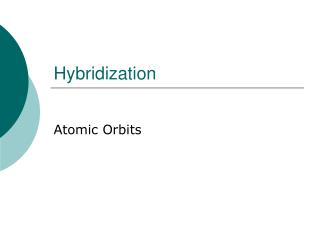# Hybridization - PowerPoint PPT PresentationDownload PresentationHybridization

HybridizationDownload Presentation## Hybridization

- - - - - - - - - - - - - - - - - - - - - - - - - - - E N D - - - - - - - - - - - - - - - - - - - - - - - - - - -
##### Presentation Transcript

1. Hybridization Atomic Orbits

2. Hybridization • the concept of mixing atomic orbitals to form new hybrid orbitals suitable for the qualitative description of atomic bonding properties

3. The sp hybrid atomic orbitals • The sp hybrid atomic orbitals are possible states of electron in an atom, especially when it is bonded to others. These electron states have half 2s and half 2p characters

4. For example, the molecule H-Be-H is formed due to the overlapping of two 1s orbitals of 2 H atoms and the two sp hybridized orbitals of Be. Thus, the H-Be-H molecule is linear. The diagram here shows the overlapping of AOs in the molecule H-Be-H

5. The sp2 hybrid orbitals • The energy states of the valence electrons in atoms of the second period are in the 2s and 2p orbitals. If we mix two of the 2p orbitals with a 2s orbital, we end up with three sp2 hybridized orbitals. These three orbitals lie on a plane, and they point to the vertices of a equilateral triangle as shown here.

6. When the central atom makes use of sp2 hybridized orbitals, the compound so formed has a trigonal shape. BF3 is such a molecule:

7. Mixing one s and all three p atomic orbitals produces a set of four equivalent sp3 hybrid atomic orbitals. The four sp3 hybrid orbitals points towards the vertices of a tetrahedron, as shown here in this photograph The sp3 hybrid orbitals

8. In the case of methane, the three 2p orbitals of the carbon atom are combined with its 2s orbital to form four new orbitals called "sp3" hybrid orbitals. • .

9. Hybridization Involving Multiple Bonds • Only a maximum of two electrons can occupy any orbital whether it is an atomic orbital or a molecular orbital due to electron-electron repulsion.

10. When we draw a double or a triple-bond between two atoms, we imply that either four or six electrons are directly between these two atoms. • Since this is impossible, we must have these extra electrons off to the side in what we refer to as pi bonds.

11. Therefore, all multiple bonds are composed of two different kinds of molecular bonds called pi-bonds and sigma-bonds.

12. The sigma-bond is defined as the linear overlap of atomic orbitals (hybrids except for hydrogen) in which two electrons are directly between the two bonded nuclei.

13. Pi-bonds are defined as the parallel overlap of p-orbitals. A double bond has one sigma-bond and one pi-bond. A triple bond thus consists of a sigma-bond and two pi-bonds with the pi-bonds in different planes